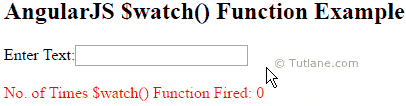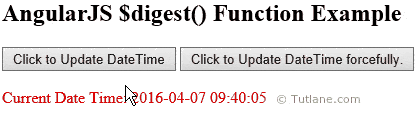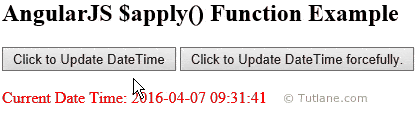# AngularJS \$watch(), \$digest() and \$apply() Functions

Here we will learn \$watch(), \$digest() and \$apply() functions in angularjs and why we need to use \$watch(), \$digest() and \$apply() functions in angularjs applications  with example.

## AngularJS Central Functions (\$watch(), \$digest(), \$apply)

In angularjs \$scope object is having different functions like \$watch(), \$digest() and \$apply() and we will call these functions as central functions.

The angularjs central functions \$watch(), \$digest() and \$apply are used to bind data to variables in view and observe changes happening in variables.

Generally in angularjs we use \$scope object to bind data to variables and use that variables values wherever we required in application. In angularjs whatever the variables we assigned with \$scope object will be added to watch list by using \$scope.\$watch() function.

In angularjs once variables added to watch list \$scope.digest() function will iterates through the watch list variables and check if any changes made for that variables or not. In case if any changes found for that watch list variables immediately corresponding event listener function will call and update respective variable values with new value in view of application.

The \$scope.\$apply() function in angularjs is used whenever we want to integrate any other code with angularjs. Generally the \$scope.\$apply() function will execute custom code and then it will call \$scope.\$digest() function forcefully to check all watch list variables and update variable values in view if any changes found for watch list variables.

In most of the time angularjs will use \$scope.watch() and \$scope.digest() functions to check and update values based on the changes in variable values.

We will learn angularjs \$watch(), \$digest, \$apply() central functions one by one in detail.

## AngularJS \$watch() Function

In angularjs \$watch() function is used to watch the changes of variables in the \$scope object. Generally in angularjs the \$watch() function will be created internally to handle variable changes in application. The \$watch() function will be useful whenever we want to create custom watch for any events.

Following is the example of using \$watch() function in angularjs applications.

<!DOCTYPE html>

<html>

<title>

AngularJs \$watch() Function with Example

</title>

<script type="text/javascript">

var app = angular.module('watchApp', []);

app.controller('watchCtrl', function (\$scope) {

\$scope.count = -1;

\$scope.\$watch('txtval', function (newval, oldval) {

\$scope.count = \$scope.count + 1;

});

});

</script>

<body>

<div ng-app="watchApp" ng-controller="watchCtrl">

<h2>AngularJS \$watch() Function Example</h2>

Enter Text:<input type="text" ng-model="txtval" /><br /><br />

<span style="color:Red">No. of Times \$watch() Function Fired: {{count}}</span>

</div>

</body>

</html>

If you observe above code we are counting how many times \$watch() function called in angularjs applications.

## Output of AngularJS \$watch() Function Example

Following is the result of using \$watch() function in angularjs applications.## AngularJS \$digest() Function

In angularjs \$digest() function is a central function of \$scope object and it is used to iterate through watch list items and check if any variable value has been modified or not. Generally in angularjs whenever we create variables along with \$scope object those variables will be added to the watch list.

In angularjs if \$digest() function found any change in watch list variables then corresponding event listener functions will call and update those values in view with new values.

Following is the example of using \$digest() function in angularjs applications.

<!DOCTYPE html>

<html>

<title>

AngularJs \$digest() Function with Example

</title>

<script type="text/javascript">

var app = angular.module('digestApp', []);

app.controller('digestCtrl', function (\$scope) {

\$scope.currentDateTime = new Date();

\$scope.updatedtime = function () {

\$scope.currentDateTime = new Date();

}

var event = document.getElementById("btndigest");

//get dateTime

\$scope.currentDateTime = new Date();

//The digest method is use to update date time forcefully.

\$scope.\$digest();

});

});

</script>

<body>

<div ng-app="digestApp" ng-controller="digestCtrl">

<h2>AngularJS \$digest() Function Example</h2>

<input type="button" value="Click to Update DateTime" ng-click="updatedtime()" />

<input type="button" value="Click to Update DateTime forcefully." id="btndigest" />

<br /><br />

<span style="color : Red">Current Date Time: {{currentDateTime | date:'yyyy-MM-dd HH:mm:ss'}}</span>

</div>

</body>

</html>

If you observe above code we added \$scope.\$digest() function to update data binding in btndigest event listener click. In case if we remove \$scope.\$digest() function in btndigest event listener click the \$scope.currentDateTime variable value will update but that new value will not reflect in view in angularjs applications.

## Output of AngularJS \$digest() Function

Following is the result of using \$digest() function in angularjs applications.## AngularJs \$apply() function

In angularjs the \$apply() function is used to evaluate expressions outside of angularjs context (like browser DOM events, setTimeout, XHR or third party libraries). Generally in angularjs once \$apply() function execution finishes forcefully it will call \$digest() function to update all data bindings.

Following are the differences between \$apply and \$digest() functions in angularjs.

1. The \$apply() and \$digest() methods are used to update the model properties forcefully.
2. The \$digest() method evaluate watchers for current scope but \$apply() method is used to evaluate watchers for root scope that means it's for all scopes.

Following is the example of using \$apply() function in angularjs applications.

<!DOCTYPE html>

<html>

<title>

AngularJs \$apply() Function with Example

</title>

<script type="text/javascript">

var app = angular.module('applyApp', []);

app.controller('applyCtrl', function (\$scope) {

\$scope.currentDateTime = new Date();

\$scope.updatedtime = function () {

\$scope.currentDateTime = new Date();

}

var event = document.getElementById("btnapply");

//The \$apply method is use to update date time on rootScope forcefully.

\$scope.\$apply(function () {

//get dateTime

\$scope.currentDateTime = new Date();

});

});

});

</script>

<body>

<div ng-app="applyApp" ng-controller="applyCtrl">

<h2>AngularJS \$apply() Function Example</h2>

<input type="button" value="Click to Update DateTime" ng-click="updatedtime()" />

<input type="button" value="Click to Update DateTime forcefully." id="btnapply" />

<br /><br />

<span style="color:Red">Current Date Time: {{currentDateTime | date:'yyyy-MM-dd HH:mm:ss'}}</span>

</div>

</body>

</html>

If you observe above code we are forcefully updating expression (\$scope.currentDateTime) value by calling \$apply() function on button click otherwise the changes whatever we made for expression (\$scope.currentDateTime) will not reflect in view of angularjs applications.

## Output of AngularJS \$apply() Function()

Following is the result of using \$apply() function in angularjs applications.This is how we can use \$watch(), \$digest() and \$apply() functions in angularjs applications.